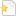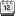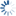## Civil Engineering

CE
Climbin GATE Institute

#HeadlinesCourse Content ( Subject Details) GATE / ESE Civil Engineering (CE)

"All the following Subjects are divided in to Chapters "

CE 1 : Engineering Mechanics :

Chapterwise Theory with Formulas and Important Tips + Chapter wise GATE /ESE Level Practice Q & A + Complete Solution

CE 2 : Solid Mechanics :

Chapterwise Theory with Formulas and Important Tips + Chapter wise GATE /ESE Level Practice Q & A + Complete Solution

CE 3 : Structural Analysis :

Chapterwise Theory with Formulas and Important Tips + Chapter wise GATE /ESE Level Practice Q & A + Complete Solution

CE 4 : Construction Materials & Management :

Chapterwise Theory with Formulas and Important Tips + Chapter wise GATE /ESE Level Practice Q & A + Complete Solution

CE 5 : Concrete Structures :

Chapterwise Theory with Formulas and Important Tips + Chapter wise GATE /ESE Level Practice Q & A + Complete Solution

CE 6 : Steel Structures :

Chapterwise Theory with Formulas and Important Tips + Chapter wise GATE /ESE Level Practice Q & A + Complete Solution

CE 7 : Soil Mechanics :

Chapterwise Theory with Formulas and Important Tips + Chapter wise GATE /ESE Level Practice Q & A + Complete Solution

CE 8 : Fluid Mechanics and Hydraulics :

Chapterwise Theory with Formulas and Important Tips + Chapter wise GATE /ESE Level Practice Q & A + Complete Solution

CE 9 : Hydrology :

Chapterwise Theory with Formulas and Important Tips + Chapter wise GATE /ESE Level Practice Q & A + Complete Solution

CE 10 : Foundation Engineering :

Chapterwise Theory with Formulas and Important Tips + Chapter wise GATE /ESE Level Practice Q & A + Complete Solution

CE 11 : Irrigation :

Chapterwise Theory with Formulas and Important Tips + Chapter wise GATE /ESE Level Practice Q & A + Complete Solution

CE 12 : Highway Engineering :

Chapterwise Theory with Formulas and Important Tips + Chapter wise GATE /ESE Level Practice Q & A + Complete Solution

CE 13 : Surveying :

Chapterwise Theory with Formulas and Important Tips + Chapter wise GATE /ESE Level Practice Q & A + Complete Solution

CE 14 : Environmental Engineering :

Chapterwise Theory with Formulas and Important Tips + Chapter wise GATE /ESE Level Practice Q & A + Complete Solution

Quick Link to Pay#Next course eventsManager(s) for CE : Climbin GATE Institute
Administrator for GATE Study Material ,GATE /IES Postal Study Notes, GATE IES Printed PDF Course, GATE Test Series and Mock Tests CS, ECE, EE, ME, CE : Team climbin
Phone : +91-9756506852